F_Futamura

•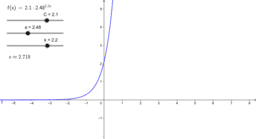Parameters of an Exponential Function

Activity

F_Futamura

•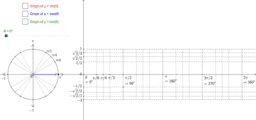Trigonometric functions and the unit circle

Activity

F_Futamura

•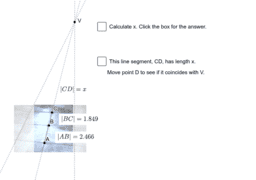Using the harmonic ratio to find the vanishing point

Activity

F_Futamura

•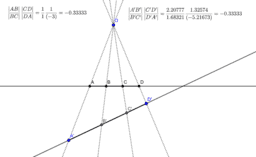Invariance of the cross ratio

Activity

F_Futamura

•7.2.1 Desargues's Theorem Proof Step 1

Activity

F_Futamura

•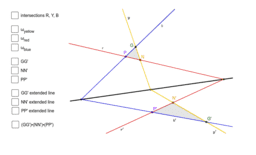7.2.2 Desargues's Theorem Step 2

Activity

F_Futamura

•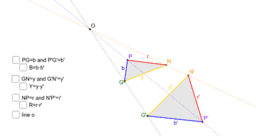7.1 Desargues's Theorem Demo

Activity

F_Futamura

•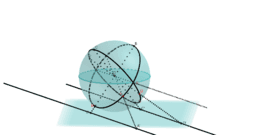Half sphere model of projective plane

Activity

F_Futamura

•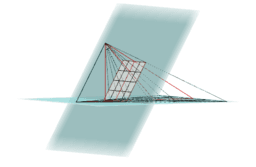Activity

F_Futamura